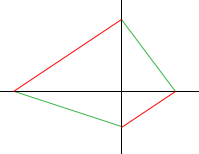SEARCH HOMEMath Central Quandaries & QueriesQuestion from Amanda, a student: Are the diagonals of a trapezoid ever perpendicular?Amanda,

Try to construct one.

Draw a pair of perpendicular lines (black).Draw a line that intersects the two black lines (red). Draw another red line parallel to the first so that the point where the two black lines intersect is between the red lines. Draw two lines to complete the quadrilateral (green).

HarleyMath Central is supported by the University of Regina and The Pacific Institute for the Mathematical Sciences.